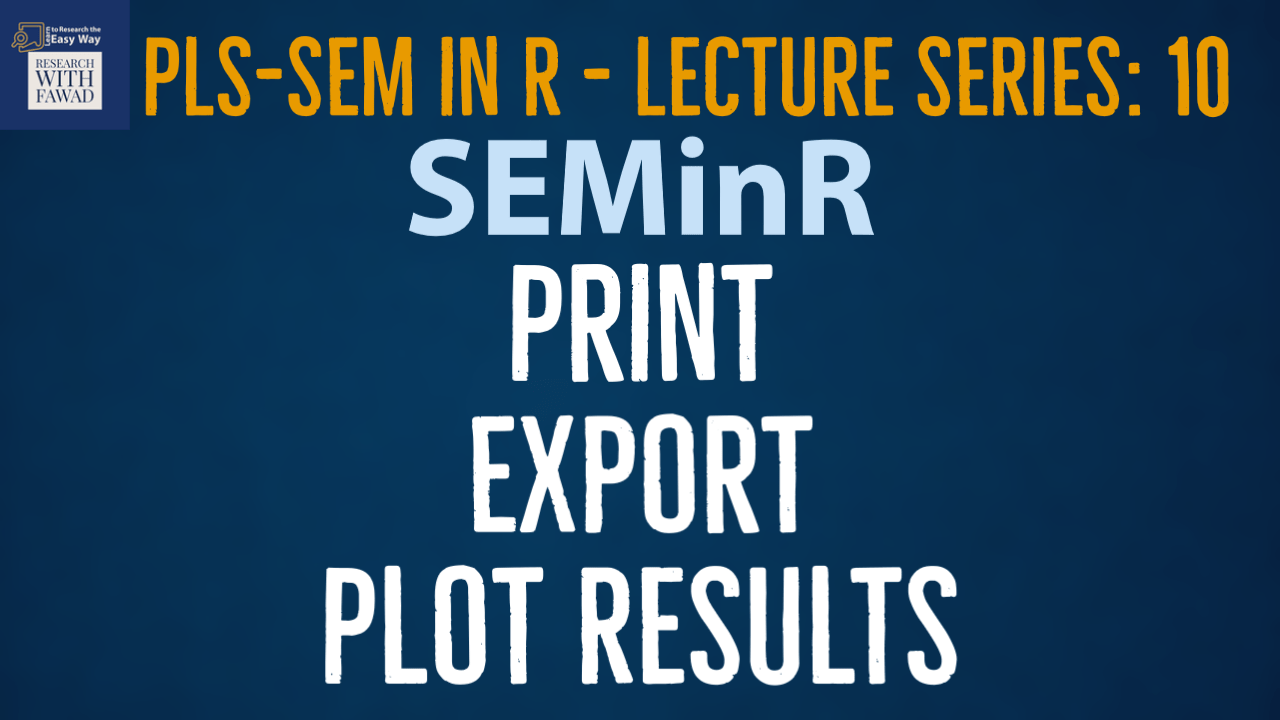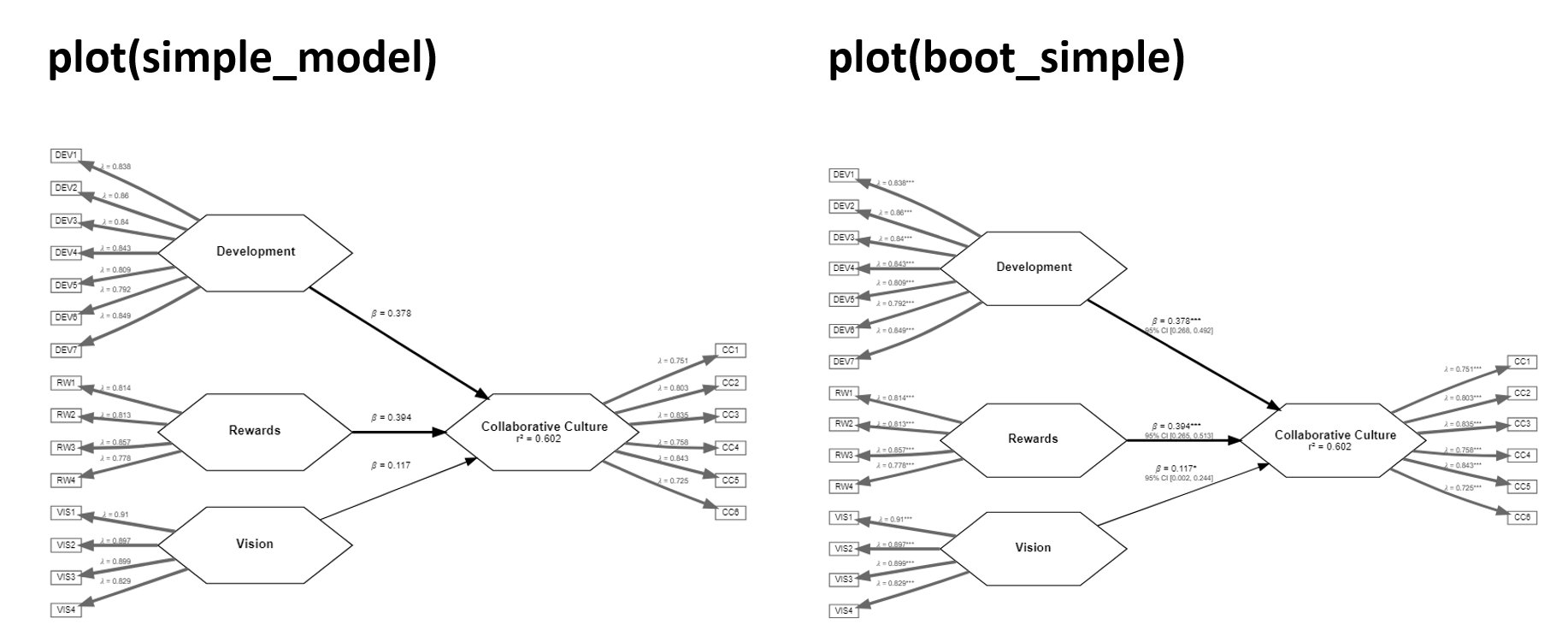# Print, Export, and Plot PLS Results in SEMinR### Learn to Print, Export, and Plot PLS Results

This series of lecture on SEMinR Package will introduce the SEMinR package.

## SEMinR

There are four steps to specify and estimate a structural equation model using SEMinR:

• Specifying the measurement models
• Specifying the structural model
• Estimating, bootstrapping, and summarizing the model

## Review of the Steps

• When model estimation, evaluation, and analysis have been completed, it is often necessary to export the results generated in R to a report. It is recommended that tables and matrices are exported to .csv files.
• The write.csv() function takes an object and writes it into a .csv file in the working directory of the project.
• This function applies two arguments: x is the name of the object to be written to file, and file is the name of the file to be created and written to.
• Thus, if we wish to report the bootstrapped paths from the previously discussed simple model, we would use the write.csv() function with argument x = summary_boot\$bootstrapped_loadings and file = “boot_loadings.csv”.

## Plots

• When plotting the results, use plot() function as shown in the figure below with command plot (summary_simple\$reliability) to extract the reliability plot.
• Next, we discuss how to generate high-quality figures from the SEMinR results.
• First, we generate a sample plot for export from RStudio. To do this, we use a sub-object of summary_simple and plot the constructs’ internal consistency reliabilities (i.e., Cronbach’s alpha, rhoA, and rhoC) with

plot(summary_simple\$reliability)

• Once this plot displays in the plots tab in RStudio (Fig), click the Export dropdown list and select Save as PDF to bring up the save plot as a .pdf window. Select the size and output name and save the document.

## Measurement and Structural Model in SEMinR

• When plotting the results, use plot() function as shown in the figure below.• In simple terms in the plot function provide, simple_model or boot_model as arguments. Both are the estimated models. simple_model is the estimated pls model whereas boot_model is the bootstrapped model.

## Review of the Steps

Following is a brief review of the steps that have been discussed in SEMinR tutorials.

• Load the Library – library ()
• Review the Data – head()
• Specify the Measurement Model – constructs()
• Specify the Structural Model – relationships()
• Estimate the Model – estimate_pls()
• Summarize the Results – summary()
• Bootstrap the Model – bootstrap_model()
• Summarize the Results – summary()

The next step is Plotting and Writing Results – plot() and Write.csv

## Complete Code

``````#load the library
library(seminr)
# Create measurement model
simple_mm <- constructs(
composite("Vision", multi_items("VIS", 1:4)),
composite("Development", multi_items("DEV", 1:7)),
composite("Rewards", multi_items("RW",1:4)),
composite("Collaborative Culture", multi_items("CC", 1:6)))
# Create structural model
simple_sm <- relationships(
paths(from = c("Vision", "Development", "Rewards"), to = "Collaborative Culture"))
# Estimate the model
simple_model <- estimate_pls(data = datas,
measurement_model = simple_mm,
structural_model = simple_sm)
# Summarize the model results
summary_simple <- summary(simple_model)
#Retreive Full Summary
summary_simple
# Inspect the model path coefficients and the R^2 values
summary_simple\$paths
# Inspect the construct reliability metrics
summary_simple\$reliability
# Bootstrap the model
boot_simple <- bootstrap_model(seminr_model = simple_model,
nboot = 1000,
cores = NULL,
seed = 123)
# Store the summary of the bootstrapped model
summary_boot <- summary(boot_simple)
#Retreive Full Report
summary_boot
# Inspect the bootstrapped structural paths
summary_boot\$bootstrapped_paths
#Generating Plots
plot(simple_model)
plot(summary_simple\$reliability)
plot(boot_simple)
# Write the bootstrapped paths object to csv file